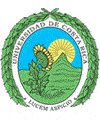``` Universidad de Costa Rica Escuela de Ciencias de la Computación e Informática ```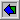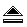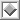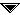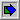# `HeapSort()`

 ```(1) for x on list L do (2) INSERT(x, S); (3) while not EMPTY(S) do begin (4) y := MIN(S); (5) writeln(y); (6) DELETE(y, S) end ```

Fig. 8.16. An abstract sorting algorithm.

 ```procedure pushdown ( first, last: integer ); { assumes A[first], . . . ,A[last] obeys partially ordered tree property except possibly for the children of A[first]. The procedure pushes A[first] down until the partially ordered tree property is restored } var r: integer; { indicates the current position of A[first] } begin r := first; { initialization } while r <= last div 2 do if last = 2*r then { r has one child at 2*r } if A[r].key > A[2*r].key then begin swap(A[r], A[2*r]); r := last { forces a break from the while-loop } end else { r has two children, elements at 2*r and 2*r + 1 } if A[r].key > A[2*r].key and A[2*r].key <= A[2*r + l].key then begin { swap r with left child } swap(A[r], A[2*r]); r := 2*r end else if A[r].key > A[2*r + l].key and A[2*r + l].key < A[2*r].key then begin { swap r with right child } swap(A[r], A[2*r + 1]); r := 2*r + l end else { r does not violate partially ordered tree property } r := last { to break while-loop } end; { pushdown } ```

Fig. 8.17. The procedure pushdown.

 ``` procedure heapsort; { sorts array A, . . . ,A[n] into decreasing order } var i: integer; { cursor into A } begin { establish the partially ordered tree property initially } (1) for i := n div 2 downto 1 do (2) pushdown(i, n ); (3) for i := n downto 2 do begin (4) swap(A, A[i]); { remove minimum from front of heap } (5) pushdown(1, i - 1) { re-establish partially ordered tree property } end end; { heapsort } ```

Fig. 8.18. The procedure heapsort.

Aho, Alfred V.; Hopcroft, John E.; Ullman, Jefrrey D.
"Data Structures and Algorithms"; Addisson Wesley Publishing Co.; 1984.